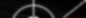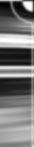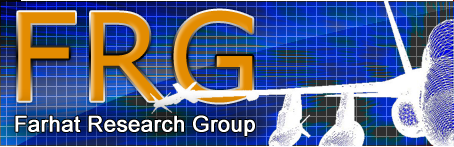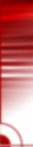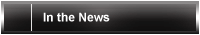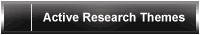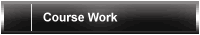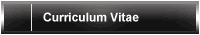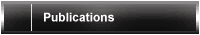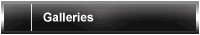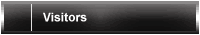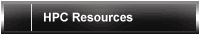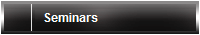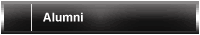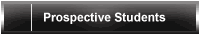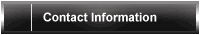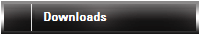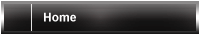DGM/DEM and Underwater Acoustics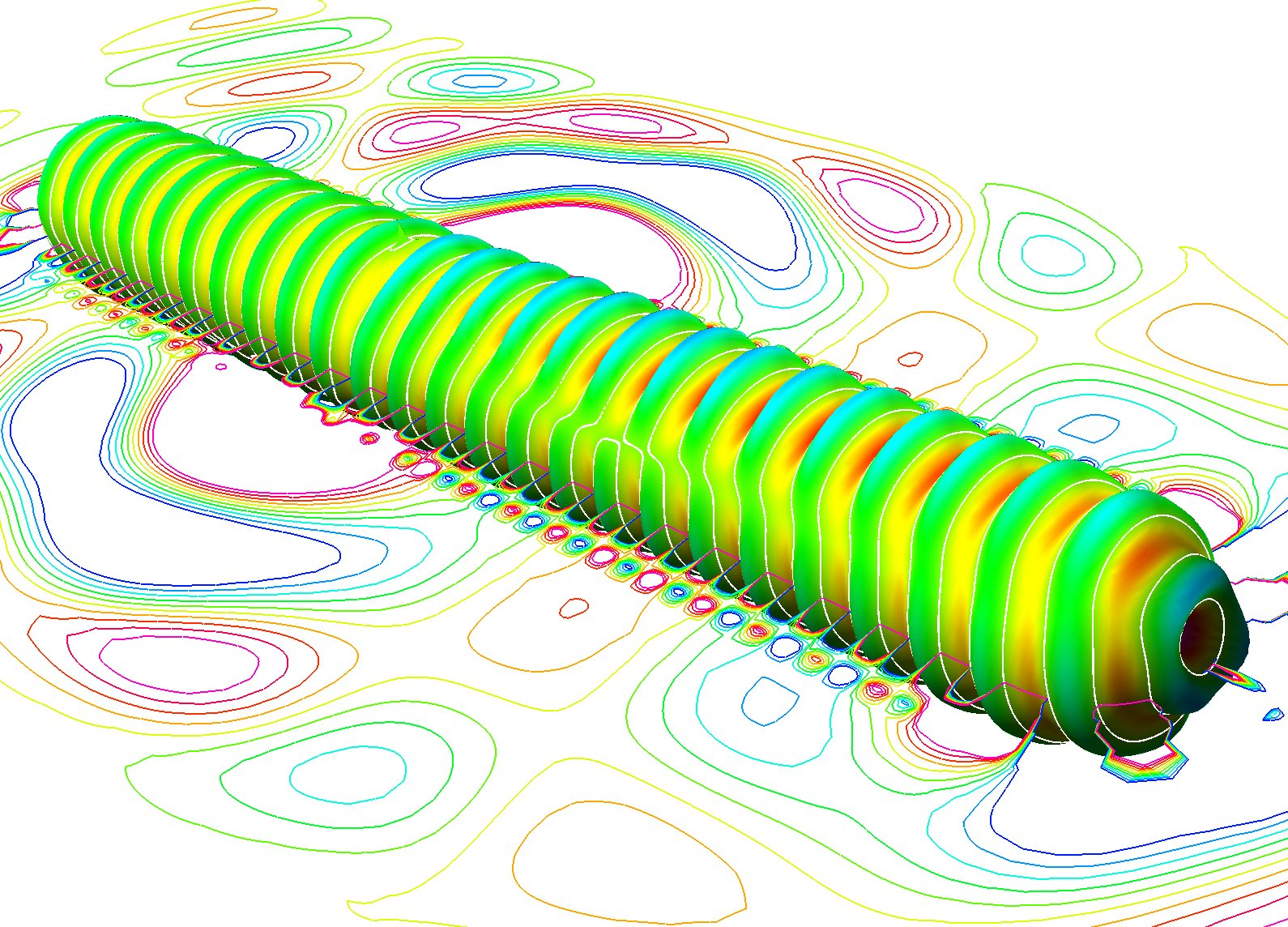Figure 1: The pressure field radiated from a point-excited capped cylindrical shell.
 Discontinuous enrichment method (DEM) is developed as a tool to solve problems of wave propagation, such as underwater scattering of acoustic waves from complex objects, and computational fluid dynamics. The method is multi-scale and significantly outperforms conventional finite element discretization in accuracy. For more information: Radek Tezaur

Computational simulation of wave propagation phenomena, for example, acoustic wave scattering and radiation from vibrating structures (Figure 1), remains one of the biggest challenges of computational mechanics. The standard FEM method is based on continuous polynomial approximations that are quasi-optimal for elliptic problems in the sense that their accuracy differs only by a factor C from that of the best approximation in the underlying finite element space. For the Helmholtz equation, which describes the acoustic vibrations of a fluid, or the Navier's equation in the frequency domain, which describes the vibrations of a solid, this factor C increases with the wavenumber. This is known as the pollution effect and it originates from the dispersive nature of the numerical waves. It also makes medium-to-high frequency simulations intractable due to their overwhelming cost since, as the frequency is increased, not only does the mesh resolution need to be increased to represent an increasing number of wavelengths in the computational domain, but also an increasing number of elements per each wavelength must be used to keep the error bounded.

DEM proposes to alleviate this difficulty by enriching the polynomial basis by functions that are relevant to the particular problem of interest. In the case of acoustic wave propagation in the fluid, a basis of uniformly distributed planar waves that solve the Helmholtz partial differential equation is used. In the case of structural vibration, transversal (shear) and longitudinal (pressure) waves are used. Computational difficulties often arise at interfaces of different materials or on the fluid-structure interface and special exponentially decaying functions can be used there to capture the so-called evanescent waves. Since scattering problems are usually posed in an infinite domain, the Sommerfeld condition that ensures that all waves are outgoing, can be, for the purpose of a finite element simulation, approximated by using the so-called perfectly matched layer (PML) and the decaying waves inside the PML are then included in the DEM basis. Work is also under way to model thin structures, such as beams, plates and shells.

As the above functions are usually discontinuous across the element boundaries, DEM uses Lagrange multipliers to ensure that the solution be continuous in a weak sense (Figure 2). This leads to a three field hybrid variational formulation for the polynomial field, the enrichment functions and the Lagrange multipliers. It has been shown for a number of application that significant gains in accuracy can be achieved over the polynomial FEM for the same cost. Alternatively, a much smaller problem is solved for the same accuracy.

Tackling the cost of the most challenging problems is possible only by developing more efficient solvers for the resulting systems of linear equations. A parallel iterative solver for the system of equations arising from a DEM formulation is being developed. The solver uses a Krylov method equipped with both a local preconditioner based on subdomain data, and a global one using a coarse space, to precondition a DEM system reduced to subdomain interfaces.

This work is funded by the U.S. Office of Naval Research under grant N00014-08-1-0184.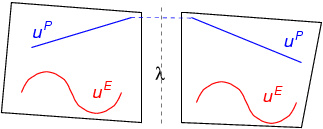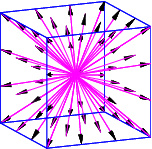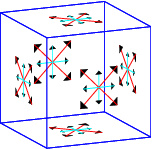Figure 2: DEM - polynomial, enrichment field and Lagrange multipliers in two elements (top); H-56-8 - hexahedral DEM element for the Helmholtz equation with 56 enrichment waves (center) and 8 Lagrange multipliers per face (bottom)